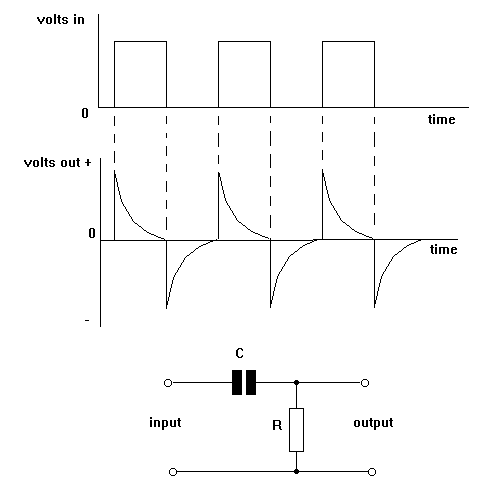Home > Electronic Tutorials > General Theory > The Differentiator Tutorial

General Theory - Electronic Tutorials

# The Differentiator Tutorial

Read the page on TIME CONSTANTS before trying this one.The differentiator is made from a capacitor C, and resistor R, and assembled as shown. A PULSE TRAIN is applied to the input.

When a pulse of voltage rises suddenly from zero to maximum, the current which is charging C suddenly rises to a maximum value as well. As C charges, the charging current falls exponentially to zero.

Since this charging current is passing through R the voltage across R (which is the output voltage) does the same. Therefore we get the shape shown, with the voltage out rising suddenly to maximum and then falling exponentially to zero.

When the pulse falls to zero C discharges. The discharge current is high at the start and then falls exponentially to zero as C discharges.

However, since the discharge current is in the opposite direction to the charge current the voltage across across R will be reversed and so the waveform is now shown below the zero line. For each pulse the waveform out is repeated giving the display shown.

Ohms Law says that current is proportional to voltage. Conversely, voltage is proportional to current.

Note: To report broken links or to submit your projects please send email to Webmaster

 Discover